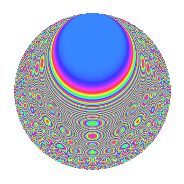# Properties

 Label 8044.2.aLevel 8044 Weight 2 Character orbit a Rep. character $$\chi_{8044}(1,\cdot)$$ Character field $$\Q$$ Dimension 167 Newforms 2 Sturm bound 2012 Trace bound 1

# Related objects

## Defining parameters

 Level: $$N$$ = $$8044 = 2^{2} \cdot 2011$$ Weight: $$k$$ = $$2$$ Character orbit: $$[\chi]$$ = 8044.a (trivial) Character field: $$\Q$$ Newforms: $$2$$ Sturm bound: $$2012$$ Trace bound: $$1$$

## Dimensions

The following table gives the dimensions of various subspaces of $$M_{2}(\Gamma_0(8044))$$.

Total New Old
Modular forms 1009 167 842
Cusp forms 1004 167 837
Eisenstein series 5 0 5

The following table gives the dimensions of the cuspidal new subspaces with specified eigenvalues for the Atkin-Lehner operators and the Fricke involution.

$$2$$$$2011$$FrickeDim.
$$-$$$$+$$$$-$$$$87$$
$$-$$$$-$$$$+$$$$80$$
Plus space$$+$$$$80$$
Minus space$$-$$$$87$$

## Trace form

 $$167q$$ $$\mathstrut -\mathstrut 4q^{5}$$ $$\mathstrut -\mathstrut 4q^{7}$$ $$\mathstrut +\mathstrut 161q^{9}$$ $$\mathstrut +\mathstrut O(q^{10})$$ $$167q$$ $$\mathstrut -\mathstrut 4q^{5}$$ $$\mathstrut -\mathstrut 4q^{7}$$ $$\mathstrut +\mathstrut 161q^{9}$$ $$\mathstrut +\mathstrut 2q^{11}$$ $$\mathstrut -\mathstrut 2q^{13}$$ $$\mathstrut -\mathstrut 8q^{15}$$ $$\mathstrut -\mathstrut 4q^{17}$$ $$\mathstrut +\mathstrut 4q^{19}$$ $$\mathstrut -\mathstrut 6q^{21}$$ $$\mathstrut -\mathstrut 4q^{23}$$ $$\mathstrut +\mathstrut 151q^{25}$$ $$\mathstrut +\mathstrut 6q^{27}$$ $$\mathstrut -\mathstrut 10q^{29}$$ $$\mathstrut -\mathstrut 10q^{31}$$ $$\mathstrut +\mathstrut 2q^{33}$$ $$\mathstrut -\mathstrut 4q^{35}$$ $$\mathstrut -\mathstrut 22q^{37}$$ $$\mathstrut -\mathstrut 20q^{39}$$ $$\mathstrut +\mathstrut 2q^{41}$$ $$\mathstrut -\mathstrut 6q^{43}$$ $$\mathstrut -\mathstrut 28q^{45}$$ $$\mathstrut -\mathstrut 16q^{47}$$ $$\mathstrut +\mathstrut 143q^{49}$$ $$\mathstrut +\mathstrut 18q^{51}$$ $$\mathstrut +\mathstrut 6q^{53}$$ $$\mathstrut -\mathstrut 18q^{55}$$ $$\mathstrut -\mathstrut 20q^{57}$$ $$\mathstrut +\mathstrut 6q^{59}$$ $$\mathstrut -\mathstrut 26q^{61}$$ $$\mathstrut -\mathstrut 22q^{63}$$ $$\mathstrut -\mathstrut 14q^{65}$$ $$\mathstrut +\mathstrut 10q^{67}$$ $$\mathstrut -\mathstrut 20q^{69}$$ $$\mathstrut +\mathstrut 14q^{71}$$ $$\mathstrut +\mathstrut 2q^{73}$$ $$\mathstrut +\mathstrut 12q^{75}$$ $$\mathstrut +\mathstrut 8q^{77}$$ $$\mathstrut -\mathstrut 36q^{79}$$ $$\mathstrut +\mathstrut 143q^{81}$$ $$\mathstrut -\mathstrut 16q^{83}$$ $$\mathstrut -\mathstrut 4q^{85}$$ $$\mathstrut +\mathstrut 2q^{87}$$ $$\mathstrut -\mathstrut 4q^{89}$$ $$\mathstrut +\mathstrut 26q^{91}$$ $$\mathstrut +\mathstrut 36q^{95}$$ $$\mathstrut +\mathstrut 2q^{97}$$ $$\mathstrut +\mathstrut 4q^{99}$$ $$\mathstrut +\mathstrut O(q^{100})$$

## Decomposition of $$S_{2}^{\mathrm{new}}(\Gamma_0(8044))$$ into irreducible Hecke orbits

Label Dim. $$A$$ Field CM Traces A-L signs $q$-expansion
$$a_2$$ $$a_3$$ $$a_5$$ $$a_7$$ 2 2011
8044.2.a.a $$80$$ $$64.232$$ None $$0$$ $$-13$$ $$-2$$ $$-12$$ $$-$$ $$-$$
8044.2.a.b $$87$$ $$64.232$$ None $$0$$ $$13$$ $$-2$$ $$8$$ $$-$$ $$+$$

## Decomposition of $$S_{2}^{\mathrm{old}}(\Gamma_0(8044))$$ into lower level spaces

$$S_{2}^{\mathrm{old}}(\Gamma_0(8044)) \cong$$ $$S_{2}^{\mathrm{new}}(\Gamma_0(2011))$$$$^{\oplus 3}$$$$\oplus$$$$S_{2}^{\mathrm{new}}(\Gamma_0(4022))$$$$^{\oplus 2}$$